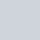Latest MCQs
Home > MCQs > Math > Xs age is twice the average age of A B and X. As age is one half the average age of A B and X. If B is 5 years old what is the average age of A B and X?

# Xs age is twice the average age of A B and X. As age is one half the average age of A B and X. If B is 5 years old what is the average age of A B and X?

A.10
B.9
C.8
D.11